# For a production function F(KL) = K-L2 and factor prices wK-2 and WL-3 Assume that K equals 27 un...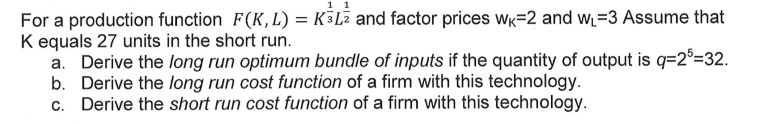For a production function F(KL) = K-L2 and factor prices wK-2 and WL-3 Assume that K equals 27 units in the short run a. Derive the long run optimum bundle of inputs if the quantity of output is q-25-32. b. Derive the long run cost function of a firm with this technology. c. Derive the short run cost function of a firm with this technology.

q = F(K, L) = K1/3L1/2

(a)

In long run, cost is minimized when MPL/MPK = wL/wK = 3/2

MPL =q/L = (1/2) x K1/3 / L1/2

MPK =q/K = (1/3) x L1/2 / K2/3

MPL/MPK = [(1/2) x K1/3 / L1/2] / [(1/3) x L1/2 / K2/3] = (3/2) x (K/L) = 3.2

K/L = 1

L = K

Substituting in production function with q = 32,

K1/3L1/2 = 32

L1/3L1/2 = 32

L5/6 = 32

Taking (6/5)th root,

L = 64

K = 64

(b)

MPL/MPK = K/L = 3/2

K = 3L/2

Substituting in generalized production function,

(3L/2)1/3L1/2 = q

(3/2)1/3L1/3L1/2 = q

1.14 x L5/6 = q

L5/6 = q / 1.14 = 0.88q

Taking (6/5)th root,

L = (0.88)6/5q6/5 = 0.85 x q6/5

K = (3/2) x L = 1.5 x 0.85 x q6/5 = 1.28 x q6/5

(c)

In short run, K = 27.

(27)1/3L1/2 = q

3L1/3L1/2 = q

3 x L5/6 = q

L5/6 = q / 3 = 0.33q

Taking (6/5)th root,

L = (0.33)6/5q6/5 = 0.27 x q6/5

K = 27

Total cost (TC) = L x wL + K x wK = 3L + 2K = 3 x (0.27 x q6/5) + 2 x 27

TC = 0.81 x q6/5 + 54

##### Add Answer of: For a production function F(KL) = K-L2 and factor prices wK-2 and WL-3 Assume that K equals 27 un...
Similar Homework Help Questions
• ### 2. Consider a firm producing pizza with production function q = KL, that faces input prices...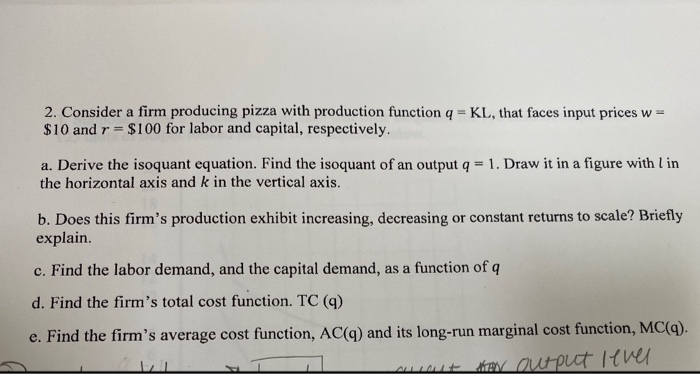2. Consider a firm producing pizza with production function q = KL, that faces input prices w= $10 and r =$100 for labor and capital, respectively. a. Derive the isoquant equation. Find the isoquant of an output q = 1. Draw it in a figure with l in the horizontal axis and k in the vertical axis. b. Does this firm's production exhibit increasing, decreasing or constant returns to scale? Briefly explain c. Find the labor demand, and the...

• ### 4. A firm produces computers with two factors of production: labor L and capital K. It's pro- duction function is ....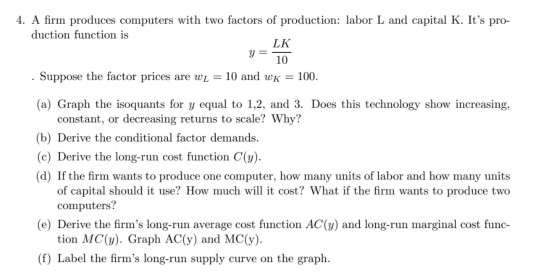4. A firm produces computers with two factors of production: labor L and capital K. It's pro- duction function is . Suppose the factor prices are wl = 10 and wK = 100. (a) Graph the isoquants for y equal to 1.2, and 3. Does this technology show increasing, constant, or decreasing returns to scale? Why? (b) Derive the conditional factor demands. (c) Derive the long-run cost function C(y). (d) If the firm wants to produce one computer, how many...

• ### 4. A firm produces computers with two factors of production: labor L and capital K. It's...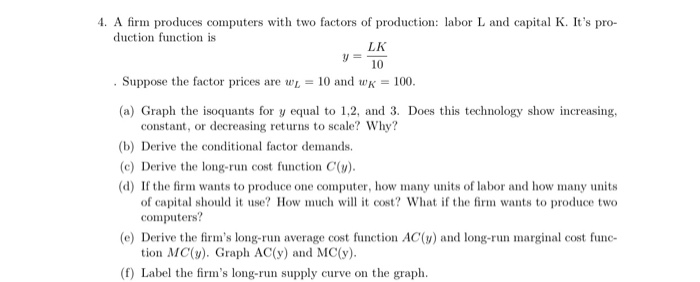4. A firm produces computers with two factors of production: labor L and capital K. It's pro- duction function is y 10 . Suppose the factor prices are wL = 10 and wk = 100. (a) Graph the isoquants for y equal to 1,2, and 3. Does this technology show increasing, constant, or decreasing returns to scale? Why? (b) Derive the conditional factor demands. (c) Derive the long-run cost function C(y). (d) If the firm wants to produce one computer,...

• ### 3. Consider the linear production function y a Br2 where aE1 and with prices w, and...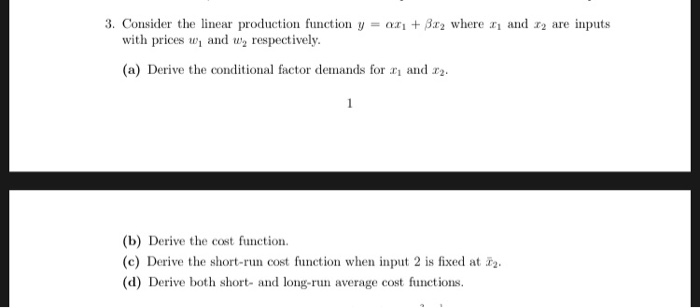3. Consider the linear production function y a Br2 where aE1 and with prices w, and w respectively are inputs (a) Derive the conditional factor demands for and . (b) Derive the cost function (c) Derive the short-run cost function when input 2 is fixed at (d) Derive both short- and long-run average cost functions.

• ### 3. [30%] Consider a competitive industry. Each firm has the following production func- tion: f (K,...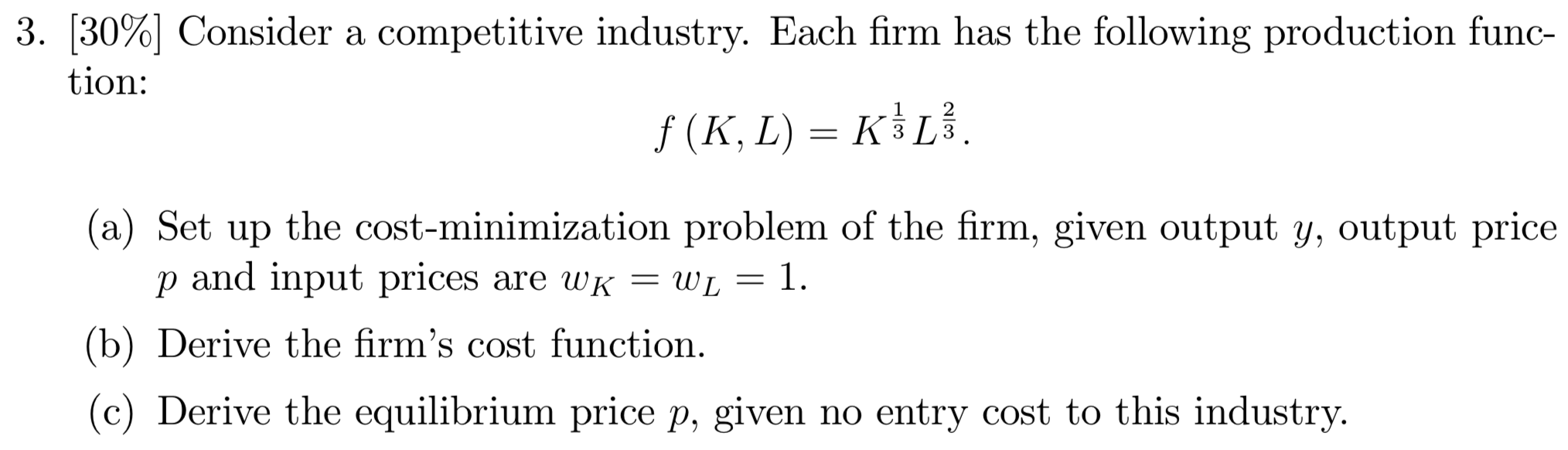3. [30%] Consider a competitive industry. Each firm has the following production func- tion: f (K, L) = K}L. WON (a) Set up the cost-minimization problem of the firm, given output y, output price p and input prices are WK = WL = 1. (b) Derive the firm's cost function. (c) Derive the equilibrium price p, given no entry cost to this industry.

• ### A firm has a production function q = KL, where q is the quantity of output,...

A firm has a production function q = KL, where q is the quantity of output, K is the amount of capital and L is the amount of labor. a) Does this production function exhibit increasing, decreasing or constant returns to scale? b) Does the long-run cost function exhibit economies of scale or diseconomies of scale? c) Is the LR Average Cost curve increasing or decreasing with q?

• ### Consider a firm using two inputs; capital (K) and labor (L) in production. The firm's production...

Consider a firm using two inputs; capital (K) and labor (L) in production. The firm's production technology is characterized by the following production function: Q = F(K, L) = 40K L In the short run (SR), the quantity of the capital (K) that the firm uses is fixed at K = 10 whereas the quantity of the labor input can be varied. Price of labor is $4,000 per worker and the price of capital is$2,000 per capital. (PL=\$4,000 and...

• ### 2. A firm has the production function y = 4LK. The marginal products are given by...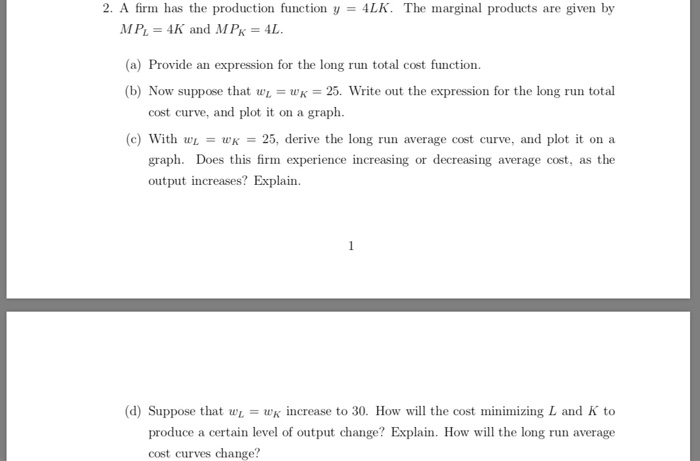2. A firm has the production function y = 4LK. The marginal products are given by MP = 4K and MPx = 4L. (a) Provide an expression for the long run total cost function. (b) Now suppose that wu = WK = 25. Write out the expression for the long run total cost curve, and plot it on a graph. (c) With WL = WK = 25, derive the long run average cost curve, and plot it on a graph....

• ### A packaging firm relies on the production function Q(L,K) = KL + L. Assuming the firm’s...

A packaging firm relies on the production function Q(L,K) = KL + L. Assuming the firm’s optimal input combination is interior (i.e. it uses positive amounts of both inputs), what is its long-run marginal cost function? a. ???? = 2√??? b. ???? = √ ?? /? c. ???? = 2√??? – r d. ???? = (2?/ √??) − r

• ### Please solve and show full work for a rating. Thank you. Plastic bags are great 2) The production of plastic bags is gi...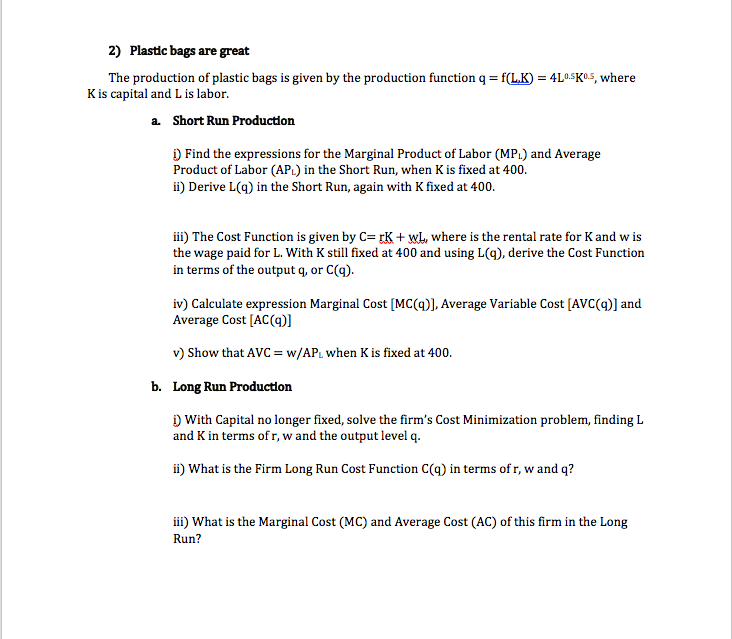Please solve and show full work for a rating. Thank you. Plastic bags are great 2) The production of plastic bags is given by the production function q K is capital and L is labor. f(LK) s, where Short Run Production a. ) Find the expressions for the Marginal Product of Labor (MP) and Average Product of Labor (APL) in the Short Run, when K is fixed at 400. i) Derive L() in the Short Run, again with K fixed...

Need Online Homework Help?